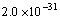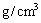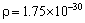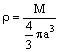(3)   Mass density

The average mass density in the universe is based on Virial mass, because comic mass refer to the mass of gravitational matter, but the property of gravitational matter is aggregation and obeying classical mechanics. Thus, it is impossible, outside the galaxy, to find matter which mass is much greater than the galaxy

The density according to a galaxy count and mass-luminosity relation is calculated atthis is universally acknowledged.

It has been calculated from coma that Virial mass is 8.3 times as great as optical mass. Therefore I adoptFormula of overage mass density is